Students can download Maths Chapter 3 Algebra Ex 3.8 Questions and Answers, Notes, Samacheer Kalvi 9th Maths Guide Pdf helps you to revise the complete Tamilnadu State Board New Syllabus, helps students complete homework assignments and to score high marks in board exams.

## Tamilnadu Samacheer Kalvi 9th Maths Solutions Chapter 3 Algebra Ex 3.8

Synthetic division calculator. This calculator divides two polynomials using synthetic division.

Question 1.
Factorise each of the following polynomials using synthetic division:
(i) x3 – 3x² – 10x + 24
Solution:
p(x) – x3 – 3x² – 10x + 24
p(1) = 13 – 3(1)² – 10(1) + 24
= 1 – 3 – 10 + 24
= 25 – 13
≠ 0
x – 1 is not a factor

p(-1) = (-1)3 – 3(-1)² – 10(-1) + 24
= – 1 – 3(1) + 10 + 24
= -1 – 3 + 10 + 24
= 34 – 4
= 30
≠ 0
x + 1 is not a factor

p(2) = 23 – 3(2)² – 10(2) + 24
= 8 – 3(4) – 20 + 24
= 8 – 12 – 20 + 24
= 32 – 32
= 0
∴ x – 2 is a factor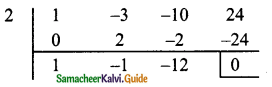x² – x – 12 = x² – 4x + 3x – 12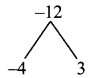= x(x – 4) + 3 (x – 4)
= (x – 4) (x + 3)
∴ The factors of x3 – 3x² – 10x + 24 = (x – 2) (x – 4) (x + 3)(ii) 2x3 – 3x² – 3x + 2
Solution:
p(x) = 2x3 – 3x² – 3x + 2
P(1) = 2(1)3 – 3(1)² – 3(1) + 2
= 2 – 3 – 3 + 2
= 2 – 6
= -4
≠ 0
x – 1 is not a factor

P(-1) = 2(-1)3 – 3(-1)² – 3(-1) + 2
= -2 – 3 + 3 + 2
= 5 – 5
= 0
∴ x + 1 is a factor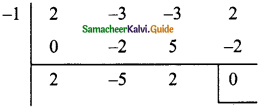2x² – 5x + 2 = 2x² – 4x – x + 2= 2x(x – 2) – 1 (x – 2)
= (x – 2) (2x – 1)
∴ The factors of 2x3 – 3x² – 3x + 2 = (x + 1) (x – 2) (2x – 1)(iii) – 7x + 3 + 4x3
Solution:
p(x) = – 7x + 3 + 4x3
= 4x3 – 7x + 3
P(1) = 4(1)3 – 7(1) + 3
4 – 7 + 3
= 7 – 7
= 0
∴ x – 1 is a factor4x² + 4x – 3 = 4x² + 6x – 2x – 3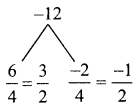= 2x(2x + 3) – 1 (2x + 3)
= (2x + 3) (2x – 1)
∴ The factors of – 7x + 3 + 4x3 = (x – 1) (2x + 3) (2x – 1)(iv) x3 + x² – 14x – 24
Solution:
p(x) = x3 + x² – 14x – 24
p(1) = (1)3 + (1)2 – 14 (1) – 24
= 1 + 1 – 14 – 24
= -36
≠ 0
x + 1 is not a factor.

p(-1) = (-1)3 + (-1)² – 14(-1) – 24
= -1 + 1 + 14 – 24
= 15 – 25
≠ 0
x – 1 is not a factor.

p(2) = (-2)3 + (-2)2 – 14 (-2) – 24
= -8 + 4 + 28 – 24
= 32 – 32
= 0
∴ x + 2 is a factor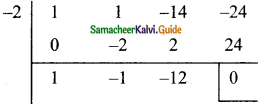x² – x – 12 = x² – 4x + 3x – 12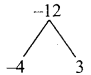= x(x – 4) + 3 (x – 4)
= (x – 4) (x + 3)
This (x + 2) (x + 3) (x – 4) are the factors.
x3 + x2 – 14x – 24 = (x + 2) (x + 3) (x – 4)(v) x3 – 7x + 6
Solution:
p(x) = x3 – 7x + 6
P( 1) = 13 – 7(1) + 6
= 1 – 7 + 6
= 7 – 7
= 0
∴ x – 1 is a factor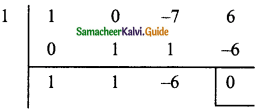x² + x – 6 = x² + 3x – 2x – 6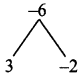= x(x + 3) – 2 (x + 3)
= (x + 3) (x – 2)
This (x – 1) (x – 2) (x + 3) are factors.
∴ x3 – 7x + 6 = (x – 1) (x – 2) (x + 3)(vi) x3 – 10x² – x + 10
p(x) = x3 – 10x2 – x + 10
= 1 – 10 – 1 + 10
= 11 – 11
= 0
∴ x – 1 is a factor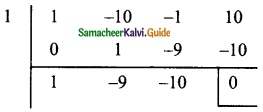x2 – 9x – 10 = x2 – 10x + x – 10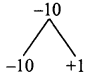= x(x – 10) + 1 (x – 10)
= (x – 10) (x + 1)
This (x – 1) (x + 1) (x – 10) are the factors.
∴ x3 – 10x2 – x + 10 = (x – 1) (x – 10) (x + 1)